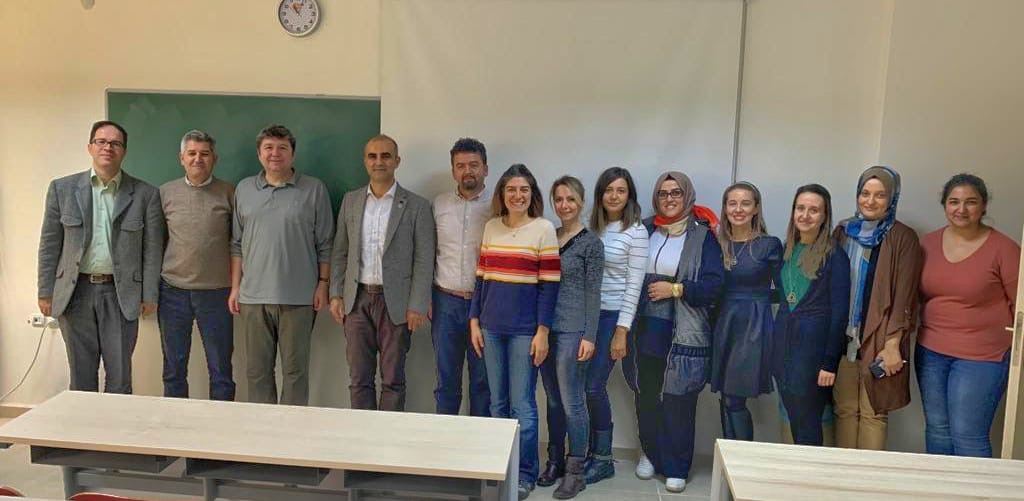Bursa Uludağ Üniversitesi
Fakülteler
Fen Edebiyat F.
Matematik Bölümü
ENG
Prof. Dr. Andrej Dujella'nın KonuşmasıZagreb Üniversitesi (Hırvatistan) Matematik Bölümü öğretim elemanı Prof. Dr. Andrej Dujella 29.11.2019 Cuma Günü Saat 14.00’ da Bölüm katındaki MAT111 No'lu Lisansüstü Dersliğinde “ Triples and quadruples which are D(n)-sets for several n's” adlı bir konuşma yapmıştır.

Katılım gösterenlere teşekkür ederiz.

Özet: For a nonzero integer n, a set of distinct nonzero integers {a1, a2, ... , am} such that ai aj + n is a perfect square for all 1 <= i < j <= m, is called a Diophantine m-tuple with the property D(n) or simply a D(n)-set. D(1)-sets are known as Diophantine m-tuples. It is natural to ask if there exists a Diophantine m-tuple (i.e. D(1)-set) which is also a D(n)-set for some n <> 1. For example, {1, 8, 120} is a D(1) and D(721)-triple. We will present infinite families of Diophantine triples {a, b, c} which are also D(n)-sets for two distinct n's with n <> 1, as  ell as some Diophantine triples which are also D(n)-sets for three distinct n's with n <> 1. We will consider similar problem with quadruples and we will show that there are infinitely many essentially different quadruples which are simultaneously D(n1)-quadruples and  (n2)-quadruples with n1 <> n2.Sosyal-Paylaşım